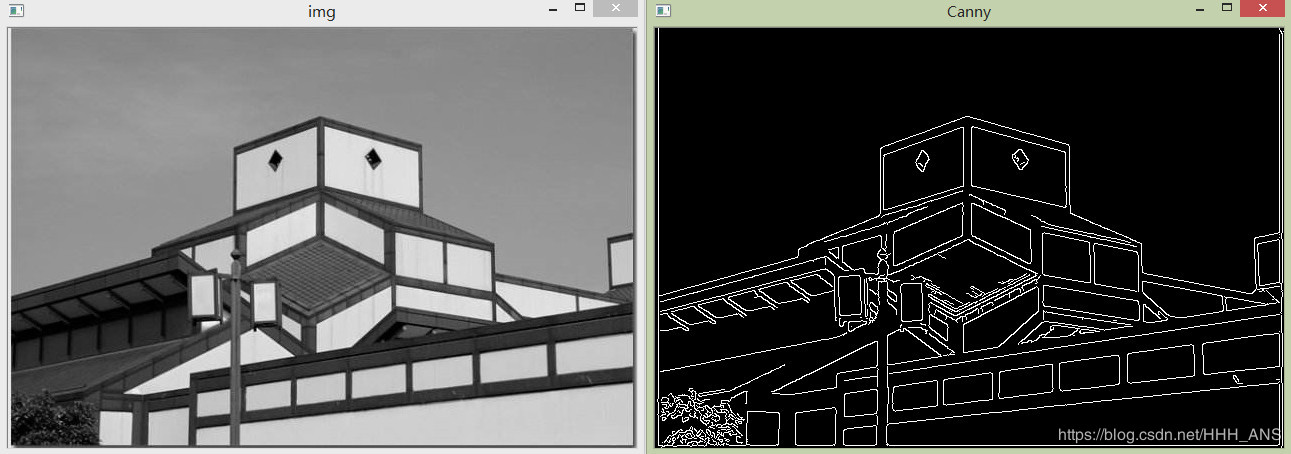# 圖像處理之邊緣檢測

### 邊緣檢測

• 邊緣檢測步驟
• 邊緣檢測基礎知識
• 邊緣模型
• 邊緣的數學概念
• 一階導數和二階導數
• 作用
• 計算一階導數
• 計算二階導數
• 邊緣檢測算子
• Roberts算子
• Prewitt算子
• Sobel算子
• 空間濾波器
• 平滑空間濾波器
• 銳化空間濾波器
• 成熟先進的邊緣檢測技術
• Marr-Hildreth邊緣檢測器
• 坎尼邊緣檢測Canny

# 邊緣檢測步驟

①濾波：邊緣檢測算法主要是基于圖像強度的一階和二階導數，但導數的計算對噪聲很敏感，因此必須使用濾波器來改善與噪聲有關的邊緣檢測器的性能。需要指出，大多數濾波器在降低噪聲的同時也導致了邊緣強度的損失，因此，增強邊緣和降低噪聲之間需要折中。
②增強:增強邊緣的基礎是確定圖像各點鄰域強度的變化值。增強算法可以將鄰域(或局部)強度值有顯著變化的點突顯出來。邊緣增強一般是通過計算梯度幅值來完成的。
③檢測：在圖像中有許多點的梯度幅值比較大，而這些點在特定的應用領域中并不都是邊緣，所以應該用某種方法來確定哪些點是邊緣點。最簡單的邊緣檢測判據是梯度幅值閾值判據。
④定位：如果某一應用場合要求確定邊緣位置，則邊緣的位置可在子像素分辨率上來估計，邊緣的方位也可以被估計出來。在邊緣檢測算法中，前三個步驟用得十分普遍。這是因為大多數場合下，僅僅需要邊緣檢測器指出邊緣出現在圖像某一像素點的附近，而沒有必要指出邊緣的精確位置或方向。

# 邊緣檢測基礎知識

### 邊緣模型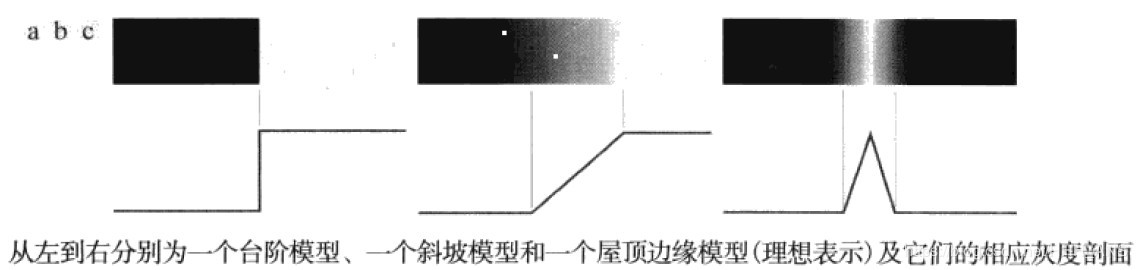### 邊緣的數學概念

1.導數，連續函數上某點斜率，導數越大表示變化率越大，變化率越大的地方就越是“邊緣”，但是在計算機中不常用，因為在斜率90度的地方，導數無窮大，計算機很難表示這些無窮大的東西。
2.微分，連續函數上x變化了dx，導致y變化了dy，dy值越大表示變化的越大，那么計算整幅圖像的微分，dy的大小就是邊緣的強弱了。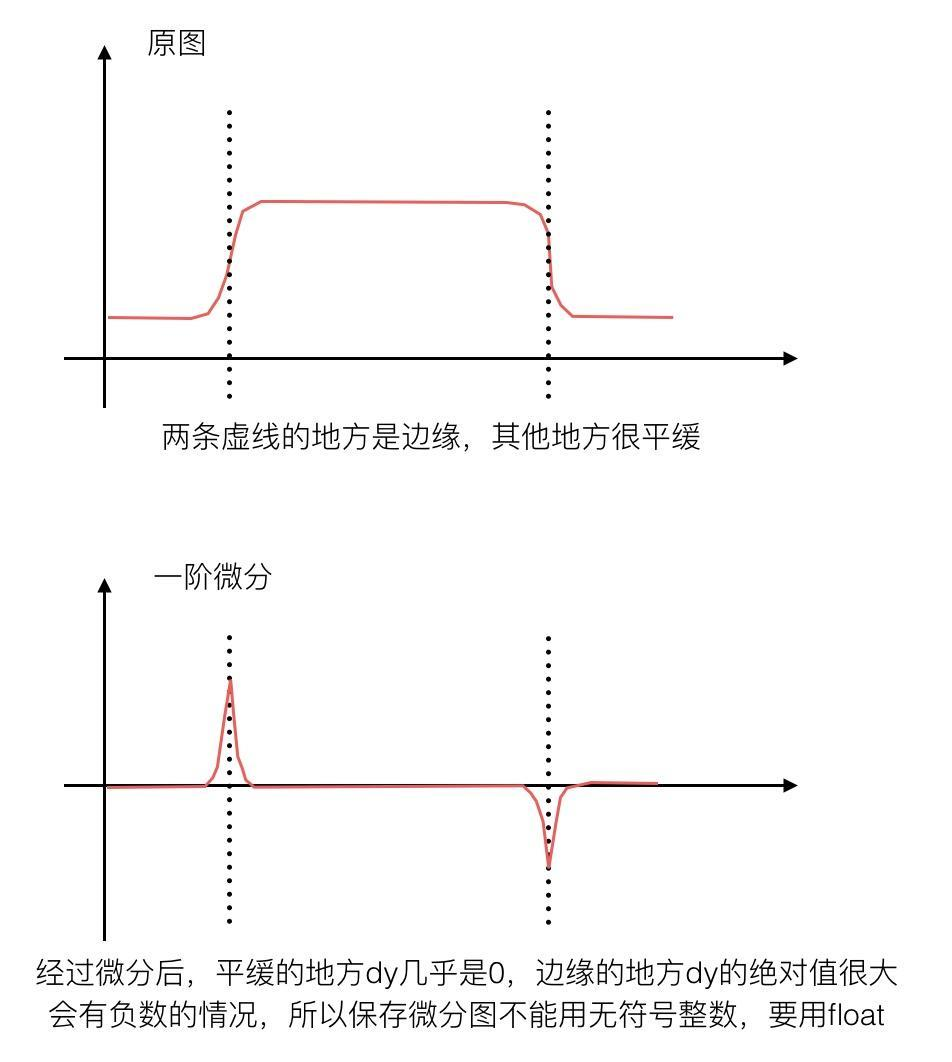Sobel邊緣檢測算子\red{Sobel 邊緣檢測算子}

Prewitt邊緣檢測算子\red{Prewitt 邊緣檢測算子}

-1, 0, 1
-1, 0, 1
-1, 0, 1
Laplacian算子\red{Laplacian算子}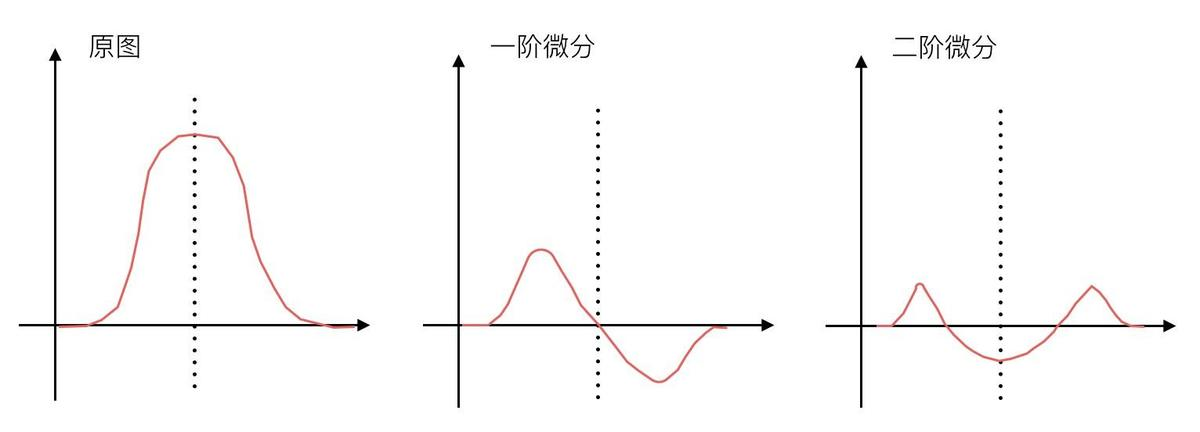f '(x, y) = -4 f(x, y) + f(x-1, y) + f(x+1, y) + f(x, y-1) + f(x, y+1)

0, 1, 0
1, -4, 1
0, 1, 0

1, 1, 1
1, -8, 1
1, 1, 1

### 一階導數和二階導數

##### 作用
• 一階導數通常在圖像中產生較粗的邊緣；
• 二階導數對精細細線，如細線、孤立點和噪聲有較強的響應；
• 二階導數在灰度斜坡和灰度臺階過渡出會產生雙邊響應；
• 二階導數的符號可以用于確定邊緣的過渡是從亮到暗還是從暗到亮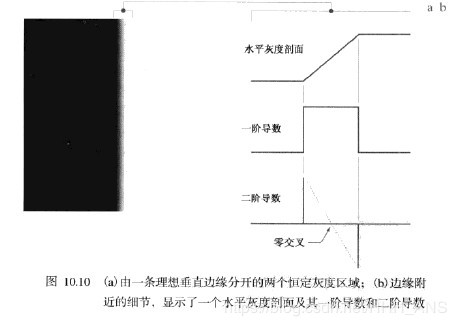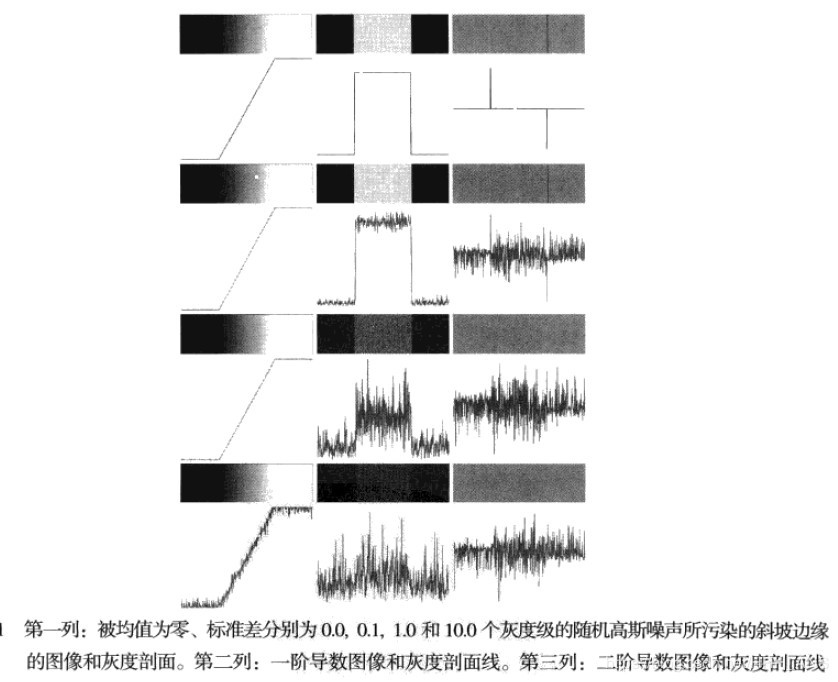##### 計算一階導數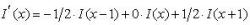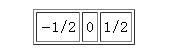##### 計算二階導數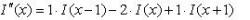### 邊緣檢測算子

##### Roberts算子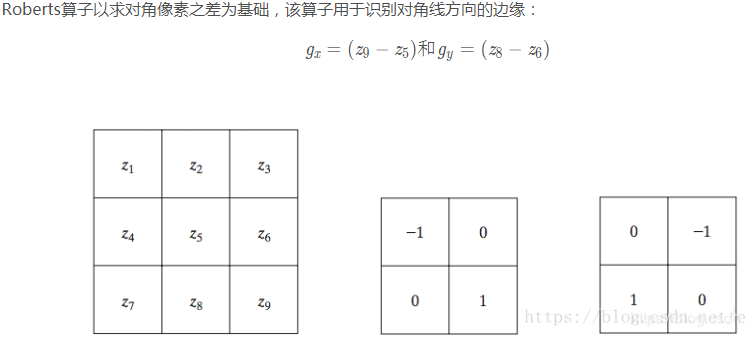##### Prewitt算子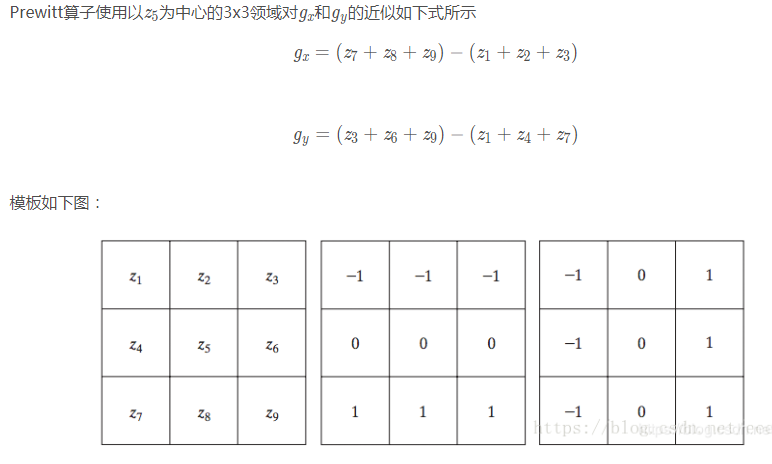##### Sobel算子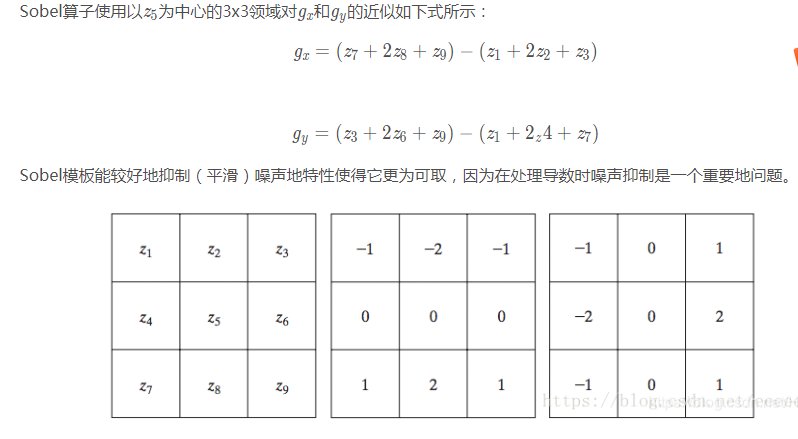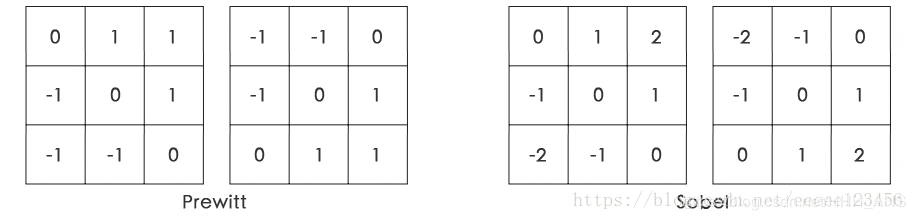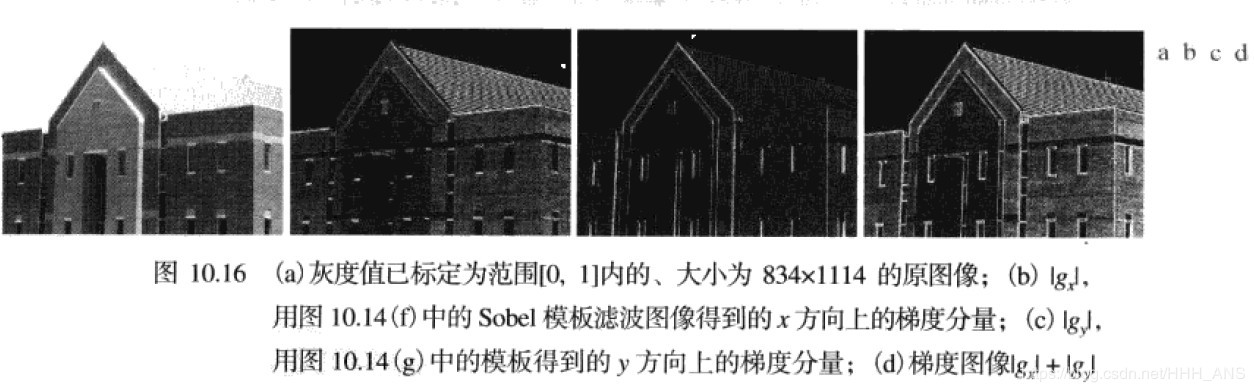### 空間濾波器

##### 平滑空間濾波器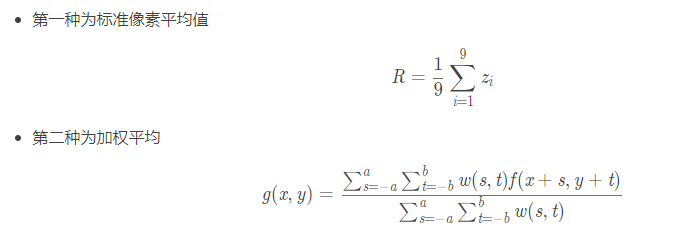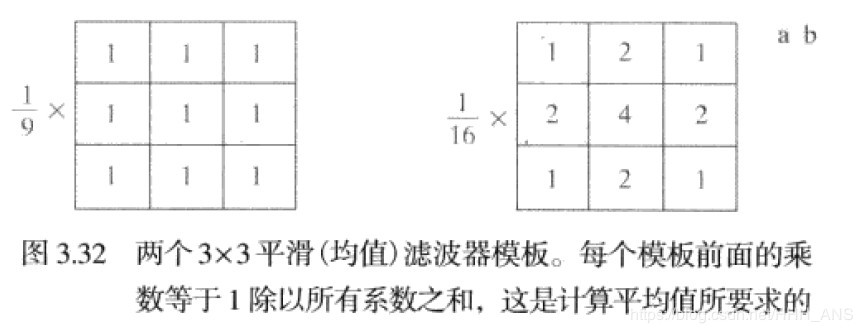import cv2
import numpy as npimg = cv2.imread('images/cat.jpg')
cv2.imshow('images',img)
"""
blur—圖像均值平滑濾波

src：圖像矩陣
ksize：濾波窗口尺寸
"""
res = cv2.blur(img, (3, 3))
cv2.imshow('res', res)
"""
GaussianBlur—圖像高斯平滑濾波

src：圖像矩陣
ksize：濾波窗口尺寸
sigmaX：標準差"""
res2 = cv2.GaussianBlur(img,(3,3),0)
cv2.imshow('res2', res2)
cv2.waitKey()import cv2
import numpy as npimg = cv2.imread('images/lena_1.jpg')
cv2.imshow('img', img)
"""
medianBlur—圖像中值濾波

src：圖像矩陣
ksize：濾波窗口尺寸"""
res = cv2.medianBlur(img, 5)
cv2.imshow('res', res)
cv2.waitKey()##### 銳化空間濾波器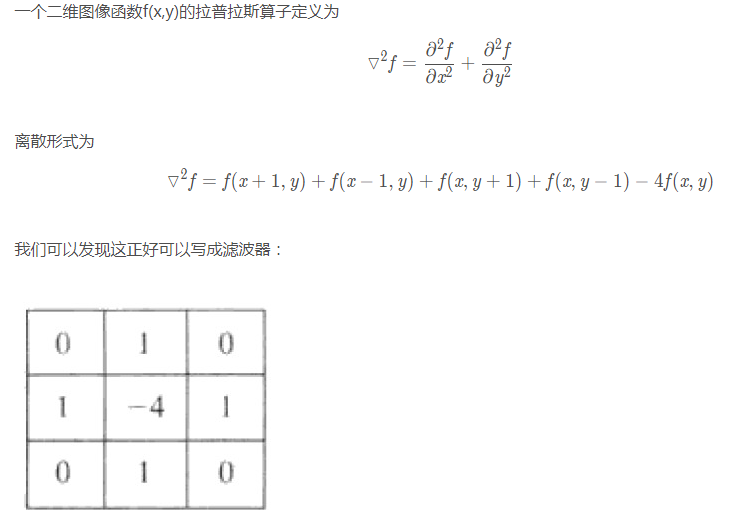import cv2
import numpy as np
cv2.imshow('img',img)
kernel = np.array([[0, -1, 0], [-1, 5, -1], [0, -1, 0]], np.float32)
#kernel = np.array([[-1,-1,-1], [-1,9,-1], [-1,-1,-1]])
dst = cv2.filter2D(img, -1, kernel=kernel)
cv2.imshow('res',dst)
cv2.waitKey(0)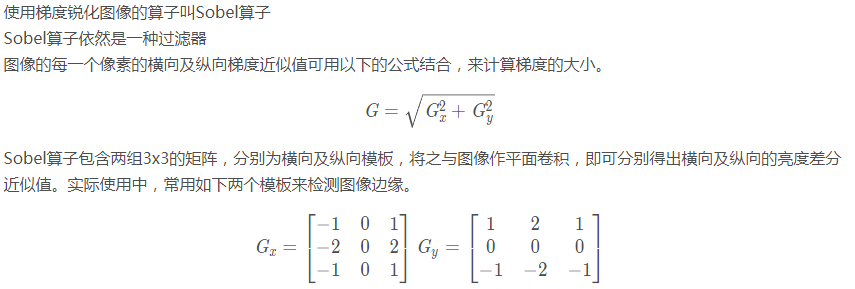# coding=utf-8
cv2.imshow('img',img)
"""
Sobel函數
Sobel(src, ddepth, dx, dy, dst=None, ksize=None, scale=None, delta=None, borderType=None)

"""
x = cv2.Sobel(img, -1, 0, 1)
cv2.imshow('res',x)
cv2.waitKey(0)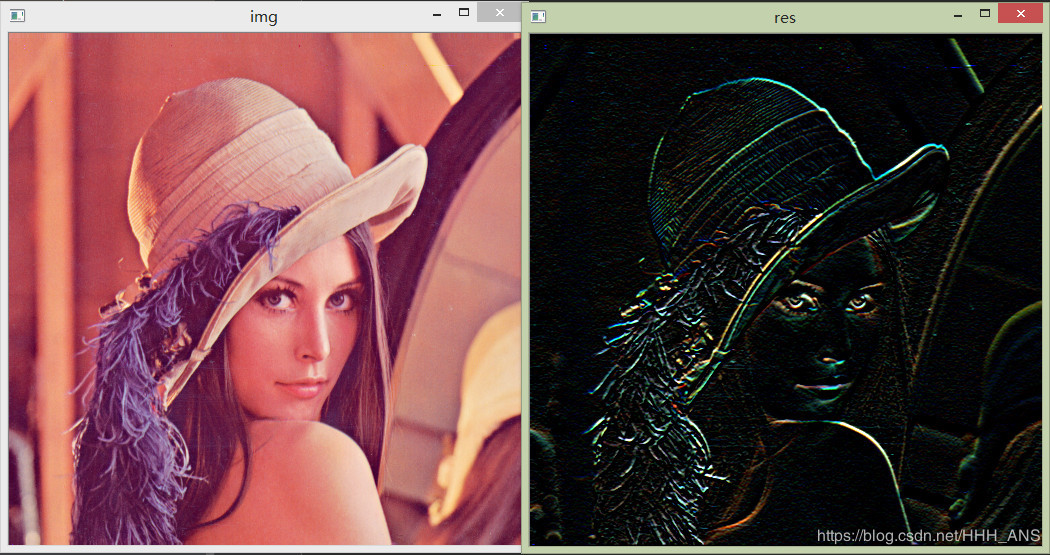# 成熟先進的邊緣檢測技術

### Marr-Hildreth邊緣檢測器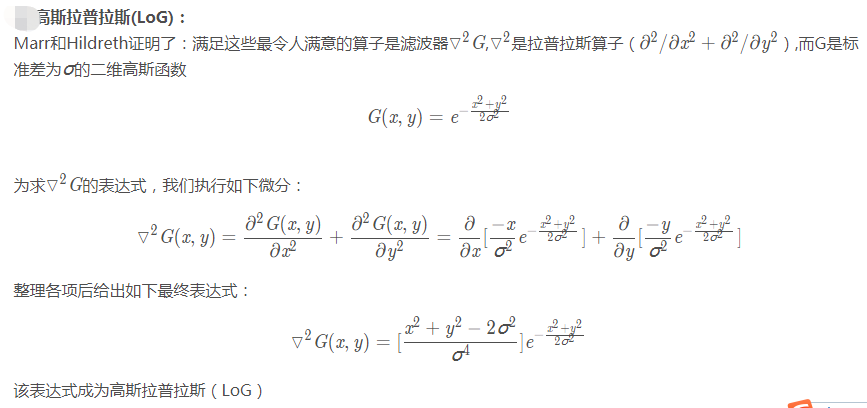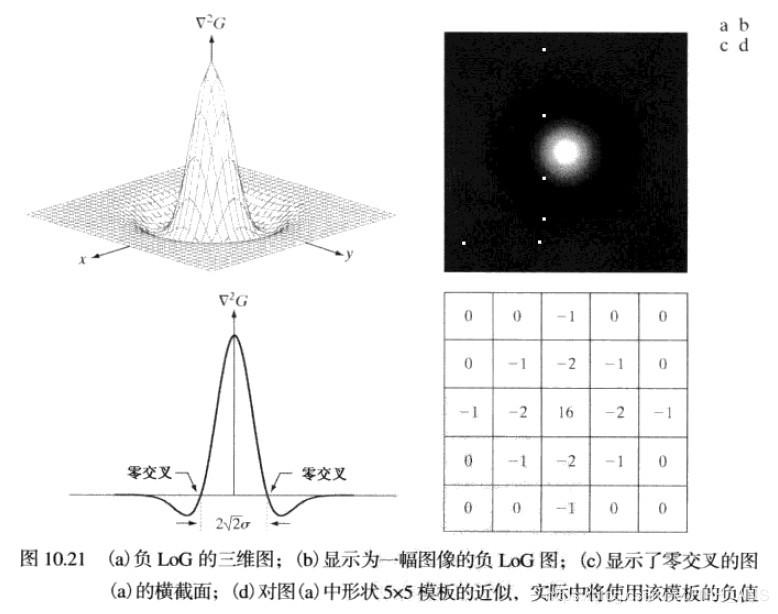Marr-Hildreth算法小結

-計算由第一步得到的圖像的拉普拉斯
-找到步驟2所有圖像的零交叉
-零交叉是Marr-Hildreth邊緣檢測方法的關鍵特征，實現簡單，并且通常能給出好的結果。

import numpy as np
import matplotlib.pyplot as plt
import cv2def edgesMarrHildreth(img, sigma):"""finds the edges using MarrHildreth edge detection method...:param im : input image:param sigma : sigma is the std-deviation and refers to the spread of gaussian:return:a binary edge image..."""size = int(2 * (np.ceil(3 * sigma)) + 1)x, y = np.meshgrid(np.arange(-size / 2 + 1, size / 2 + 1), np.arange(-size / 2 + 1, size / 2 + 1))normal = 1 / (2.0 * np.pi * sigma ** 2)kernel = ((x ** 2 + y ** 2 - (2.0 * sigma ** 2)) / sigma ** 4) * np.exp(-(x ** 2 + y ** 2) / (2.0 * sigma ** 2)) / normal  # LoG filterkern_size = kernel.shapelog = np.zeros_like(img, dtype=float)# applying filterfor i in range(img.shape - (kern_size - 1)):for j in range(img.shape - (kern_size - 1)):window = img[i:i + kern_size, j:j + kern_size] * kernellog[i, j] = np.sum(window)log = log.astype(np.int64, copy=False)zero_crossing = np.zeros_like(log)# computing zero crossingfor i in range(log.shape - (kern_size - 1)):for j in range(log.shape - (kern_size - 1)):if log[i][j] == 0:if (log[i][j - 1] < 0 and log[i][j + 1] > 0) or (log[i][j - 1] < 0 and log[i][j + 1] < 0) or (log[i - 1][j] < 0 and log[i + 1][j] > 0) or (log[i - 1][j] > 0 and log[i + 1][j] < 0):zero_crossing[i][j] = 255if log[i][j] < 0:if (log[i][j - 1] > 0) or (log[i][j + 1] > 0) or (log[i - 1][j] > 0) or (log[i + 1][j] > 0):zero_crossing[i][j] = 255# plotting imagesfig = plt.figure()a = fig.add_subplot(1, 2, 1)imgplot = plt.imshow(log, cmap='gray')a.set_title('Laplacian of Gaussian')a = fig.add_subplot(1, 2, 2)imgplot = plt.imshow(zero_crossing, cmap='gray')string = 'Zero Crossing sigma = 'string += (str(sigma))a.set_title(string)plt.show()return zero_crossingimg = cv2.imread('images/17.jpg',0)
img = edgesMarrHildreth(img,4)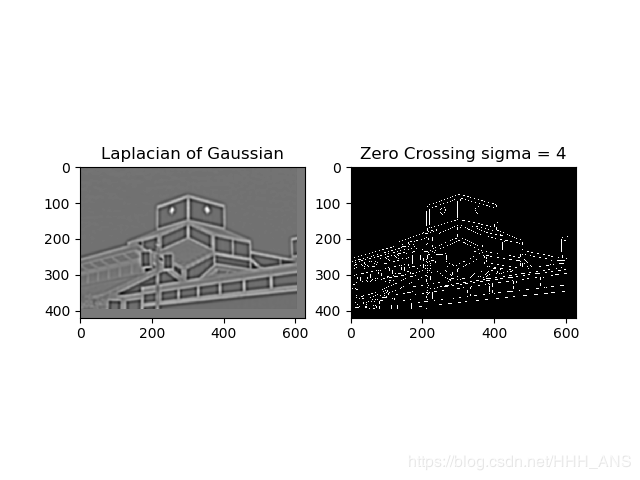### 坎尼邊緣檢測Canny

Canny目標
-低錯誤率。所有邊緣都應被找到，并且應該沒有偽相應，也就是檢測到的邊緣必須盡可能是真是的邊緣
-邊緣點應被很好的定位。已定位邊緣必須盡可能接近真實邊緣。也就是由檢測器標記為邊緣的點和真實邊緣的中心之間的距離應該最小
-單一的邊緣點響應。對于真實的邊緣點，檢測器僅應返回一個點。也就是真是邊緣周圍的局部最大數應該是最小的。這意味著在僅存一個單一邊緣點到額位置，檢測器不應指出多個邊緣像素。
Canny算法步驟
1、用一個高斯濾波器平滑輸入圖像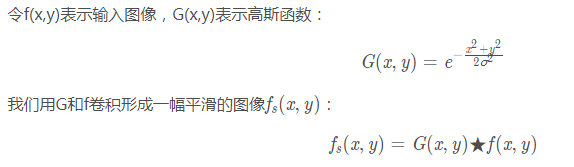2、計算梯度幅度圖像和角度圖像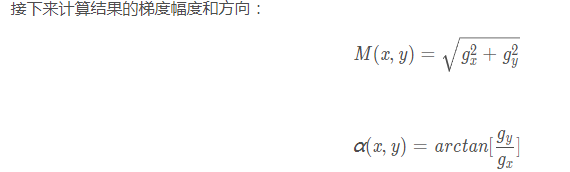3、對梯度幅度圖像應用非最大抑制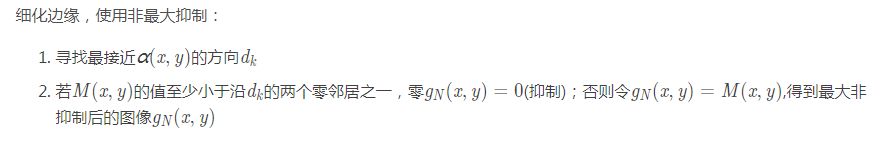4、用雙閾值處理和連接分析來檢測并連接邊緣（這相對Marr-Hildreth優化了邊緣的連接使得檢測的邊緣更加完整）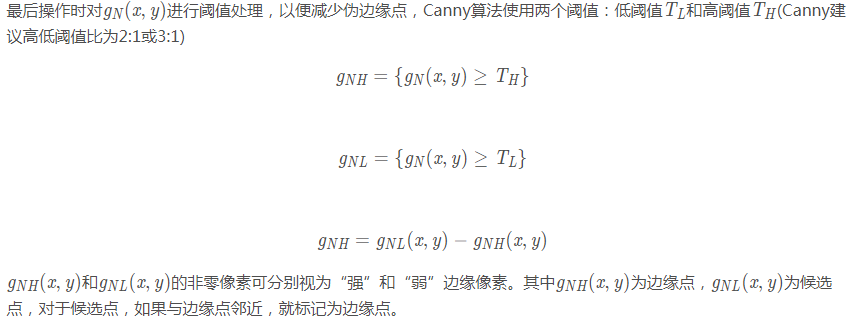# coding=utf-8
import cv2
import numpy as npimg = cv2.imread("images/17.jpg", 0)
cv2.imshow('img',img)
img = cv2.GaussianBlur(img, (3, 3), 0)
canny = cv2.Canny(img, 50, 150)cv2.imshow('Canny', canny)
cv2.waitKey(0)
cv2.destroyAllWindows()